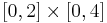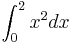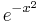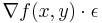# Informatics2-2020/Lab12

(eltér) ←Régebbi változat | Aktuális változat (eltér) | Újabb változat→ (eltér)

# Exercises

## Introduction

Some exercises to get used to numpy

1. Make a vector of length 10 with elements all zero! Then modify its 4th element to 1 (zeros)
2. Make a 3-by-3 matrix with elements ranging from 0 up to 8 (reshape)
3. Make a random vector of length 30 containing random number between 0 to 1! Calculate its average and standard deviation! (rand, mean, std)
1. Make a random vector of the same length with elements between -3 and 2!
4. Make a random unit vector in 5 dimensions! First make a random vector in 5 dimensions and then normalize it to unit length!

## Monte-Carlo

Generate 500000 random points in the rectangle$[0,2]\times[0,4]$. Count how many of the points (x,y) have the property that x2 > y. Use this to approximate the integral$\int_0^2x^2 d x$ Like in the end of the lecture.

## Numeric integral

Estimate the integral of$e^{-x^2}$ on the interval [ − 2,5] with the left Riemann sum!

Let's have a vector-to-scalar function f(x,y) = x2 + y2. Starting from (x0,y0) = ( − 1, − 1) we will wind the minimum of the function. A gradient step is when you subtract the$\nabla f(x,y)\cdot \epsilon$ from the (x,y) point. If you do this for small ε many times then the point will converge a point where you cannot increase the function value any more, i.e. the gradient is zero. This way you can find the minimum of the function (it will be (x,y) = (0,0)).